Published: 16 May 2022

# Simulation analysis and verification of take-up judder caused by cumulative geometric deviation of clutch friction plates

Jingchang Chen1
Ximin Yang2
Tao Zhao3
Peiran Li4
1, 4State Key Laboratory of Vehicle NVH and Safety Technology, Chongqing, China
2, 3, 1China Automotive Engineering Research Institute Co., Jinyu street, No. 9, Chongqing, China
Corresponding Author:
Jingchang Chen
Views 46

#### Abstract

In this paper, the take-up judder phenomenon caused by the accumulated geometric deviation of clutch plates is simulated and verified by experiments. Firstly, the vibration test and data analysis are carried out for the sample vehicle and related benchmark vehicles, and the vibration target is determined. Then, a simulation analysis and optimization model of the clutch engagement process in the vehicle take-up process was established, and the optimal control target was determined by multi-condition analysis of the accumulation geometric deviation factors of the clutch. Finally, several clutch deviation samples are verified, and total deviation of clutch control strategy is given. This paper provides a quantitative analysis method of the clutch cumulative geometric deviation in the take-up process, which provides a reference for the clutch parameter setting and the vibration control of the vehicle in the take-up process.

## 1. Introduction

NVH performance has become more and more important as people have higher and higher quality requirements for automobiles . As a common NVH problem, vehicle take-up judder seriously affects the comfort performance of the vehicle, and the low-frequency vibration during the take-up process is easily perceived and questioned. Judder is a phenomenon of back-and-forth vibration range 5-20 Hz, caused by the torsional vibrations of the driveline, which occur during the clutch engagement process [2-3]. There are two main reasons for the take-up judder, one is the negative gradient of the friction coefficient of the clutch friction plate which cause the self-excited vibration of the transmission system, the second is the change of the external load, such as the change of the positive pressure of the friction plate caused by the geometric deviation of clutch friction plates, and the transmission torque of clutch is change, which causes the back and forth vibration of the body.

Shangguan et al.  analyzed the influence of the torsional stiffness of the driven disk and the axial stiffness of the waveform cushion on the starting judder based on the proposed model. Sivanesan and Jayabalaji  developed a four degree of freedom torsional power train lumped mass model and observed that the clutch stick-slip is increased with increase in external torque and clutch pressure fluctuations.

In this paper, the take-up judder vibration of a certain vehicle was tested, and the vibration levels of competing vehicles were compared to determine the judder vibration control target; then the dynamic modeling during the clutch engagement process was established, and the clutch transmission torque was analyzed which is most important factor. In the simulation analysis model, the actual engine speed and torque output data are used to analyze the main vibration frequency and amplitude of vehicle; Finally, several clutches with different cumulative deviations are tested, and the control target of clutch friction cumulative deviation value is formulated according to the test results and subjective evaluation, so as to provide reference and basis for the later control of clutch friction plate deviation value.

## 2. Problem description

In the take-up process of a certain vehicle, there is a phenomenon of front and back vibration, which causes more complaints in the market. In order to analyze this problem, we arranged vibration acceleration sensors on the seat rail for objective measurement, analyzed the vibration amplitude and frequency, and evaluated the vibration level with some benchmark vehicle data. These competitive vehicles are equipped with 7-gear dual clutch transmission. During the starting process, they all have the problem of take-up judder. However, due to the different design and matching of powertrain system, they show different vibration levels in the phenomenon of take-up judder.

Due to the difference in front and back vibration frequency components of different vehicles, The Root Mean Square (RMS)value of seat rail $X$ direction vibration in 5-15 Hz was selected as a reference index for evaluation.

Fig. 1Frequency spectrum curves of take-up judder vibration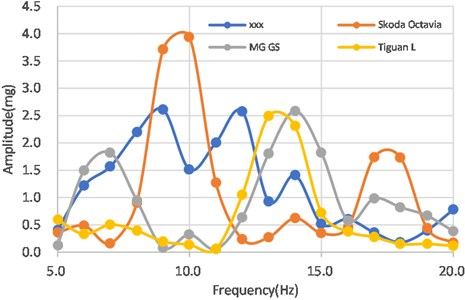Table 1Comparison of RMS value between sample vehicle and benchmark vehicles

 Vehicle type Root mean square value (mg) xxx 4.58 Skoda Octavia 4.65 MG GS 3.51 Tiguan L 3.01

## 3. Dynamic model

In order to study the influence of cumulative geometric deviation of friction plates on vehicle take-up vibration in the clutch engagement process, it is necessary to establish a torsional vibration model including engine, clutch and whole vehicle, in which all components are equivalent in the way of centralized mass. The dynamic model of take-up process is shown in Fig. 2.

Fig. 2Dynamic model for clutch engagement process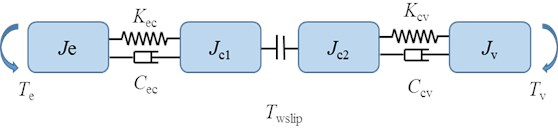In the model shown in Fig. 2: ${J}_{e}$ is the equivalent inertia of the engine crankshaft, piston and connecting rod, and ${J}_{c1}$ is the moment of inertia of the engine flywheel and clutch driving plate; ${J}_{c2}$ is the equivalent inertia of the clutch driven plate and the shaft system associated with the driven plate; ${J}_{v}$ is the equivalent inertia of the shafting and translational mass associated with the transmission output shaft; ${K}_{ec}$ and ${C}_{ec}$ are the torsional stiffness and damping between the engine crankshaft output end and the flywheel respectively; ${K}_{ev}$ and ${C}_{ev}$ are the torsional stiffness and damping from the transmission output shaft to the whole vehicle respectively; ${T}_{e}$ is the engine output torque; ${T}_{wslip}$ is the torque transmitted by the clutch friction plate; ${T}_{v}$ is the road resistance moment of the whole vehicle.

When the driving part and the driven part of the clutch are in sliding state, the dynamic equation is:

1
$\begin{array}{l}{J}_{e}{\stackrel{¨}{\theta }}_{e}+{C}_{ec}\left({\stackrel{˙}{\theta }}_{e}-{\stackrel{˙}{\theta }}_{c1}\right)+{K}_{ec}\left({\theta }_{e}-{\theta }_{c1}\right)={T}_{e},\\ {J}_{c1}{\stackrel{¨}{\theta }}_{c1}+{C}_{ec}\left({\stackrel{˙}{\theta }}_{c1}-{\stackrel{˙}{\theta }}_{e}\right)+{K}_{ec}\left({\theta }_{c1}-{\theta }_{e}\right)=-{T}_{\omega slip},\\ {J}_{c2}{\stackrel{¨}{\theta }}_{c2}+{C}_{cv}\left({\stackrel{˙}{\theta }}_{c2}-{\stackrel{˙}{\theta }}_{v}\right)+{K}_{cv}\left({\theta }_{c2}-{\theta }_{v}\right)={T}_{\omega slip},\\ {J}_{v}{\stackrel{¨}{\theta }}_{v}+{C}_{cv}\left({\stackrel{˙}{\theta }}_{v}-{\stackrel{˙}{\theta }}_{c2}\right)+{K}_{cv}\left({\theta }_{v}-{\theta }_{c2}\right)=-{T}_{v}.\end{array}$

The transmitted torque considering the negative gradient change of the friction coefficient of the clutch friction plates (Stribeck effect) is calculated as Eq. (2), and the clutch transmitted torque considering the geometric deviation of the clutch friction plates is calculated as Eq. (3):

2
${T}_{\omega slip_1}=nrF\left({u}_{0}+{u}^{\text{'}}\left({\stackrel{˙}{\theta }}_{c1}-{\stackrel{˙}{\theta }}_{c2}\right)\right),$
3
${T}_{\omega slip_2}=nru{K}_{disc}\left({X}_{0}+X*\mathrm{s}\mathrm{i}\mathrm{n}\left(\theta \right)\right),$

where, $n$ – number of clutch discs; $r$ – equivalent radius of clutch disc; $F$ – force of pressure plate; ${X}_{0}$ – nominal clutch (pressure plate) displacement; $X$ – clutch displacement variation due to geometrical deviations; ${K}_{disc}$ – axial stiﬀness of clutch disc; ${f}_{slip}$ – clutch slip speed frequency.

Hence, clutch transmitted torque is as represented by Eq. (4):

4
${T}_{\omega slip}=nr{K}_{disc}\left({X}_{0}+X*sin\left(\theta \right)\right)*\left({u}_{0}+{u}^{\text{'}}\left({\stackrel{˙}{\theta }}_{c1}-{\stackrel{˙}{\theta }}_{c2}\right)\right).$

## 4. Simulation analysis

In order to analyze the influence of clutch parameters on the take-up judder in detail, a simulation analysis model according to dynamic model of clutch engagement process is established based on 1D simulation analysis software AMESIM, as shown in Fig. 3.

In the model, the clutch transmitted torque is mainly determined by the pressure of the clutch and the friction parameters of the friction plates. The pressure of the clutch needs to consider the influence of the cumulative geometric deviation of the friction plates. Therefore, in the process of building the simulation model, it is necessary to model the relevant characteristics of the friction system of the clutch friction plates and the change of the pressure caused by the cumulative deviation of the friction plates in detail.

In the beginning of the simulation, the initial engine speed is set to 650 RPM which is the engine idle speed, the transmission gear is local in 1st position, and the clutch friction plate do not transmit torque. In the process of engagement process, with the clutch hydraulic oil pressure and the friction plates of pressure increase, the clutch friction plates start to transmit torque, and vehicles began to take up.

The influence of different geometric cumulative deviations on the amplitude of vehicle back and forth vibration during the clutch engagement process is analyzed quantitatively by the simulation model. The fast Fourier transform (FFT)analysis results of the back-and-forth vibration of the vehicle body in the clutch engagement process shows that the back and forth vibration amplitude increases linearly with the geometrical accumulation deviation of friction plates. When the geometric accumulation deviation of friction plates is equal to 0.08 mm, the vehicle vibration amplitude is 0.04 m/s2.

Fig. 3Simulation analysis model of body vibration during clutch engagement process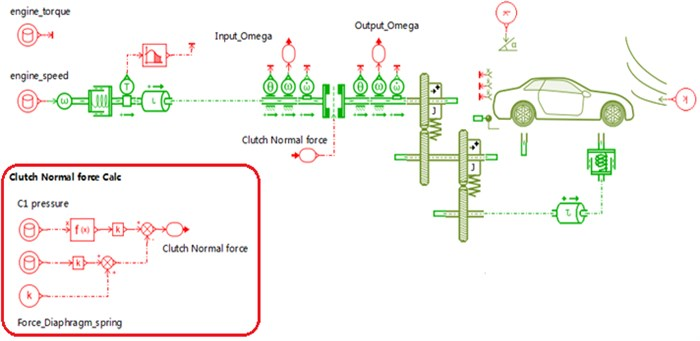Fig. 4Engine and input shaft speed curves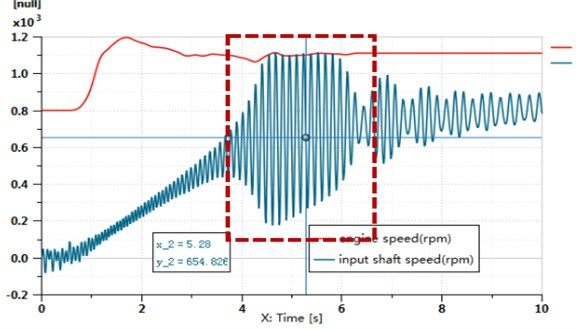Fig. 5Vehicle linear acceleration (FFT) curves of different deviations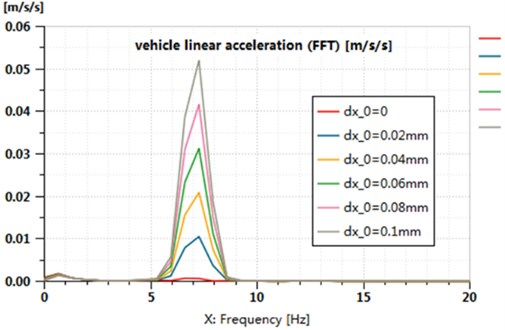## 5. Validation

This vehicle is equipped with hydraulic dual clutch transmission (DCT), in which each clutch is composed of four friction plates. The total cumulative geometric deviation value is the final result after considering the high and low matching of four friction plates. The variation of this parameter causes the fluctuation of clutch pressure force. Its fluctuation frequency is related to the difference of engine and clutch output shaft speed.

The total deviation of four clutch assemblies was measured, and the objective test was carried out on the same vehicle. The correlation coefficient between back-and-forth vibration and total deviation in the clutch engagement process was 0.793. If the back-and-forth vibration amplitude target is 4 mg, the total deviation of clutch should be controlled less than 0.08 mm.

Table 2Objective test values of different clutch assemblies

 Clutch number 5-15 Hz RMS value (mg) Total deviation value (mm) 1# 3.08 0.0522 2# 3.12 0.0465 3# 3.36 0.0681 4# 2.84 0.0486

Fig. 6Vehicle vibration RMS and clutch deviation values of different clutch assemblies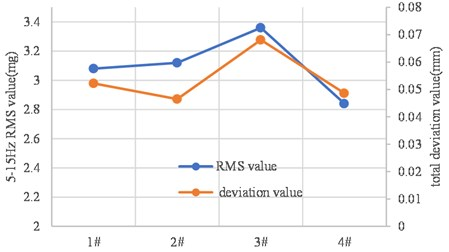Fig. 7Vehicle vibration RMS target setting-based simulation and test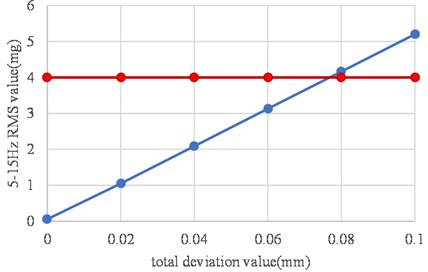## 6. Conclusions

1) The vehicle vibration in the take-up process is mainly related to the change of transmission torque during the clutch engagement process, which is mainly reflected in two aspects: the change of negative gradient of friction coefficient and the change of positive pressure of friction plates.

2) The change of positive clutch pressure is related to the cumulative geometric deviation of the clutch friction plates, and the control of the cumulative geometric deviation can effectively control the vibration level in the take-up process.